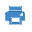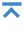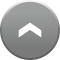﻿ Transform vector map using control points.

# Transform vector map using control points.The task «Transform vector map using control points» is intended for transformation of the plane rectangular objects coordinates of vector map. In the task two types of transformation are realized: - offset, scale, rotate; - Affine transformation. Definition of parameters of transformation is performed over coordinates of points in input and output system of coordinates.At start of the task the list of input maps is filled with the vector maps opened in a current window. At a choice of an input map from the list the name of output map automatically changes. To change automatically generated name of output map it is possible having clicked the button. If the map consists of several sheets (map) then after transformation all objects will be saved into one sheet (sit). If a name of input and output map are equal, at the included mode «Retain a copy of the original maps» before transformation the retaining an input map will be done in folder Copy_ map name_version number. After selecting the input and output map, you must select the type of transformation and catalogs of coordinates of control points. Catalog of coordinates is a text file, in which are recorded by line the number and the coordinates of points, separated by a space. The order of recording points in the catalog of coordinates has no value as at reading the automatic sorting the points by numbers is carried out. Example of the directory of input coordinates: 1 49511.17 7850.37 2 39078.21 1520.5 4 50829.666 4353.274   3 43131.266 412.382 Example of the directory of output coordinates: 3 431465.94 2188139.61 1 437739.57 2195666.78 2 427397.83 2189190.36 4 439107.24 2192188.92 The directory of coordinates can be created manually in the text editor or is received at saving the marked objects of a map into a text file in the task «Map Editor». After a choice of catalogues of coordinates the transformation coefficients, a mean-square error and residual differences on control points in output system of coordinates are automatically calculated. The process of transformation starts when you click «Run» button. If the output map already exists, the question is asked to confirm the replacement of map. After finishing the transformation the output map automatically opens in a new window. Depending on the chosen type of transformation the following formulas for transformation of coordinates are used: - offset, scale, rotate- affine transformationUnknown coefficients of transformation offset, scale, rotate are the affine transformation.,.     The unknown coefficients are determined by solving the system of normal equations. For one point it is possible to make 2 equations, therefore for transformation offset, scale, rotate it is necessary minimum of 2 points, and for affine transformation a minimum of 3 points. If number of points more than a necessary minimum the system of the equations is solved by a method of the least squares.